This book is in Open Review. I want your feedback to make the book better for you and other readers. To add your annotation, select some text and then click the on the pop-up menu. To see the annotations of others, click the button in the upper right hand corner of the page

## 13.4 Examples of application

In order to see how ADAM works on intermittent data, we consider the same example from the Section 13.1. We remember that in that example both demand occurrence and demand sizes increase over time, meaning that we can try the model with trend for both parts:

plot(y)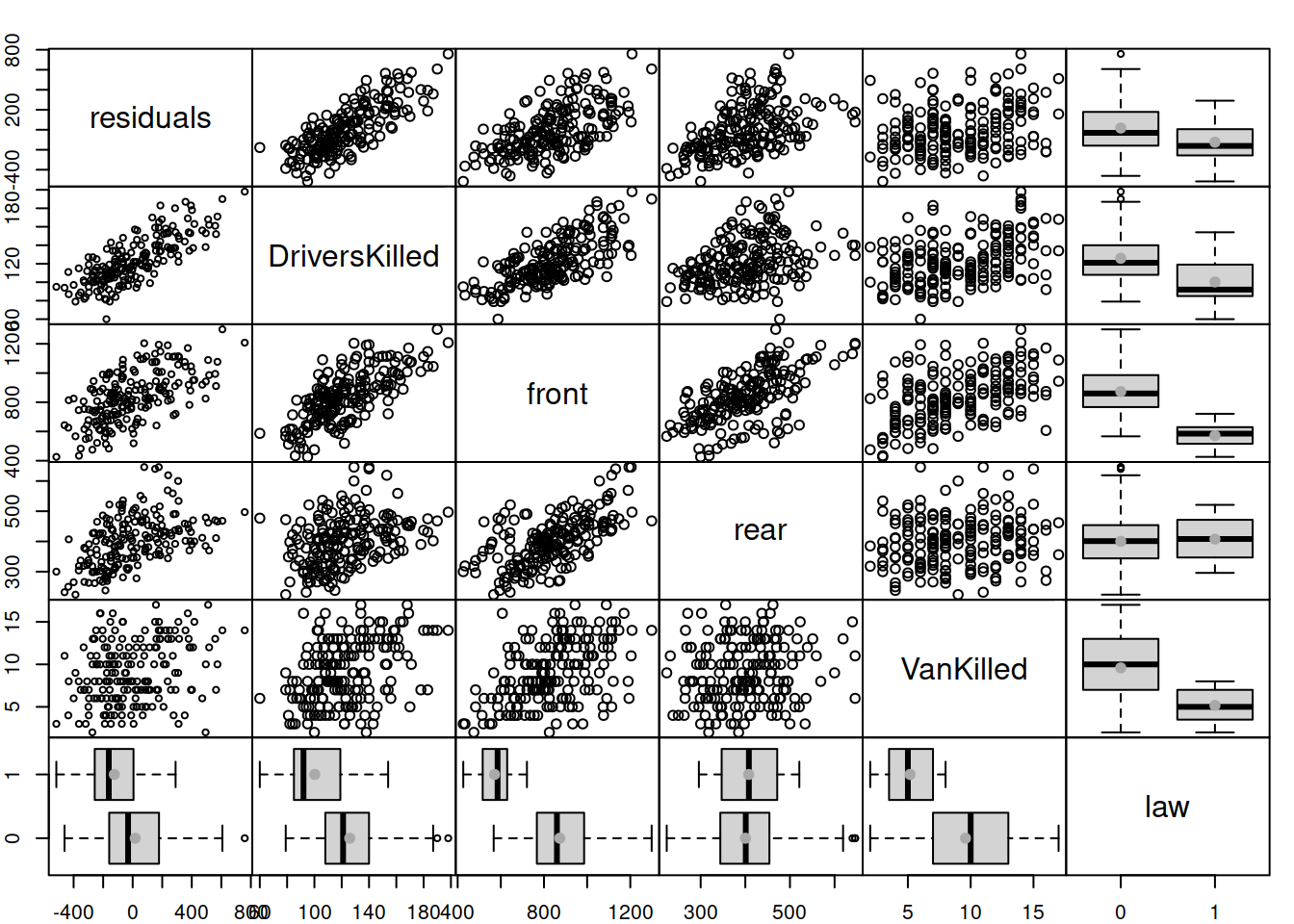This can be done using adam() function from smooth package, defining the type of occurrence to use. We will try several options and select the one that has the lowest AICc:

adamModelsiETS <- vector("list",4)
occurrence="odds-ratio")
occurrence="inverse-odds-ratio")
occurrence="direct")
occurrence="general")
c("odds-ratio", "inverse-odds-ratio",
"direct", "general"))
adamModelsiETSAICcs
##         odds-ratio inverse-odds-ratio             direct            general
##           436.0611           432.1285           433.1348           447.7490

Based on this, we can see that the model with inverse-odds-ratio has the lowest AICc. We can see how the model has approximated the data and produced forecasts for the holdout:

i <- which.min(adamModelsiETSAICcs)
plot(adamModelsiETS[[i]],7)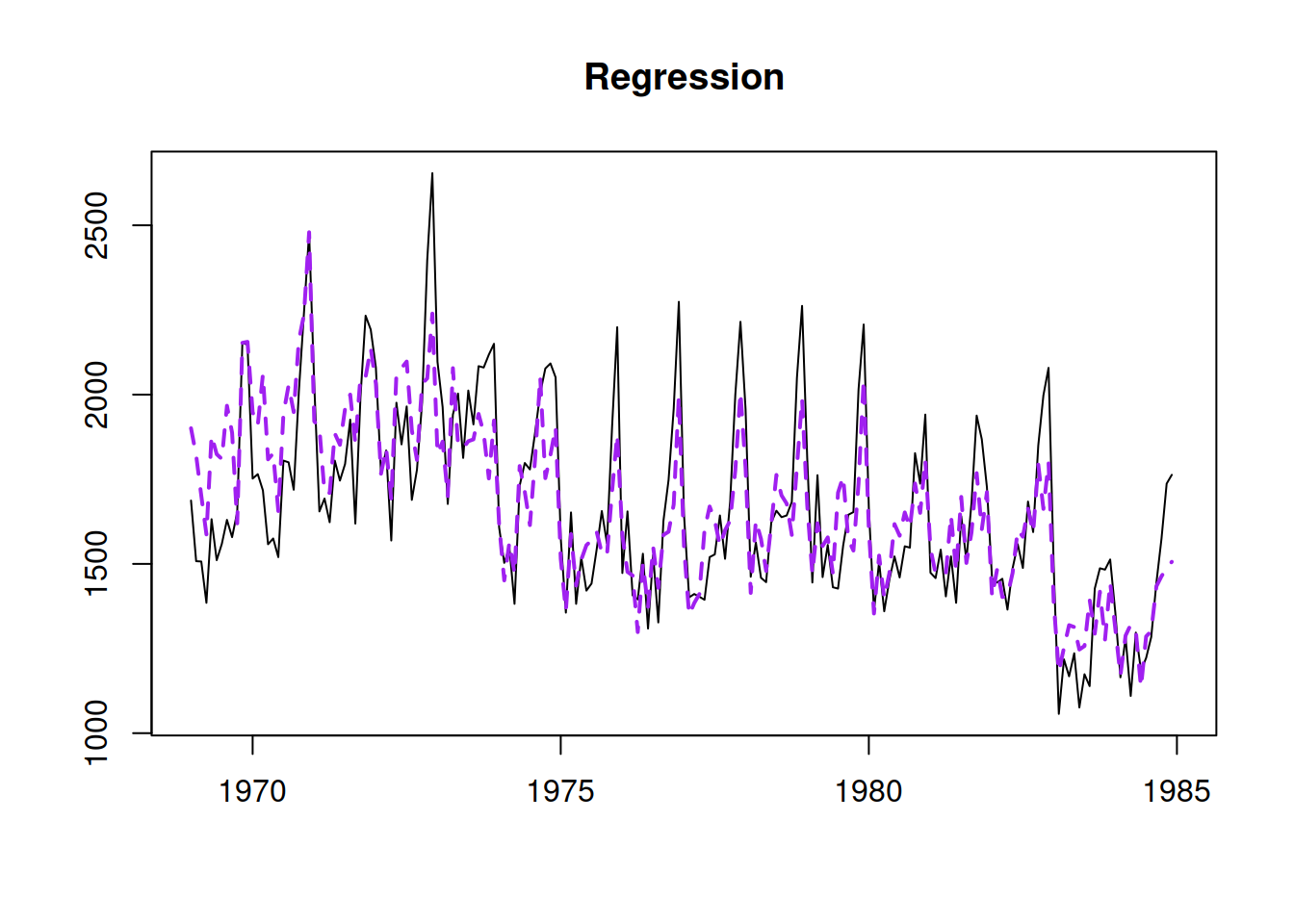We can explore the demand occurrence part of this model the following way:

adamModelsiETS[[i]]$occurrence ## Occurrence state space model estimated: Inverse odds ratio ## Underlying ETS model: oETS[I](MMdN) ## Smoothing parameters: ## level trend ## 0 0 ## Vector of initials: ## level trend ## 9.3173 0.9256 ## ## Error standard deviation: 3.0974 ## Sample size: 110 ## Number of estimated parameters: 5 ## Number of degrees of freedom: 105 ## Information criteria: ## AIC AICc BIC BICc ## 116.7930 117.3699 130.2954 131.6513 plot(adamModelsiETS[[i]]$occurrence)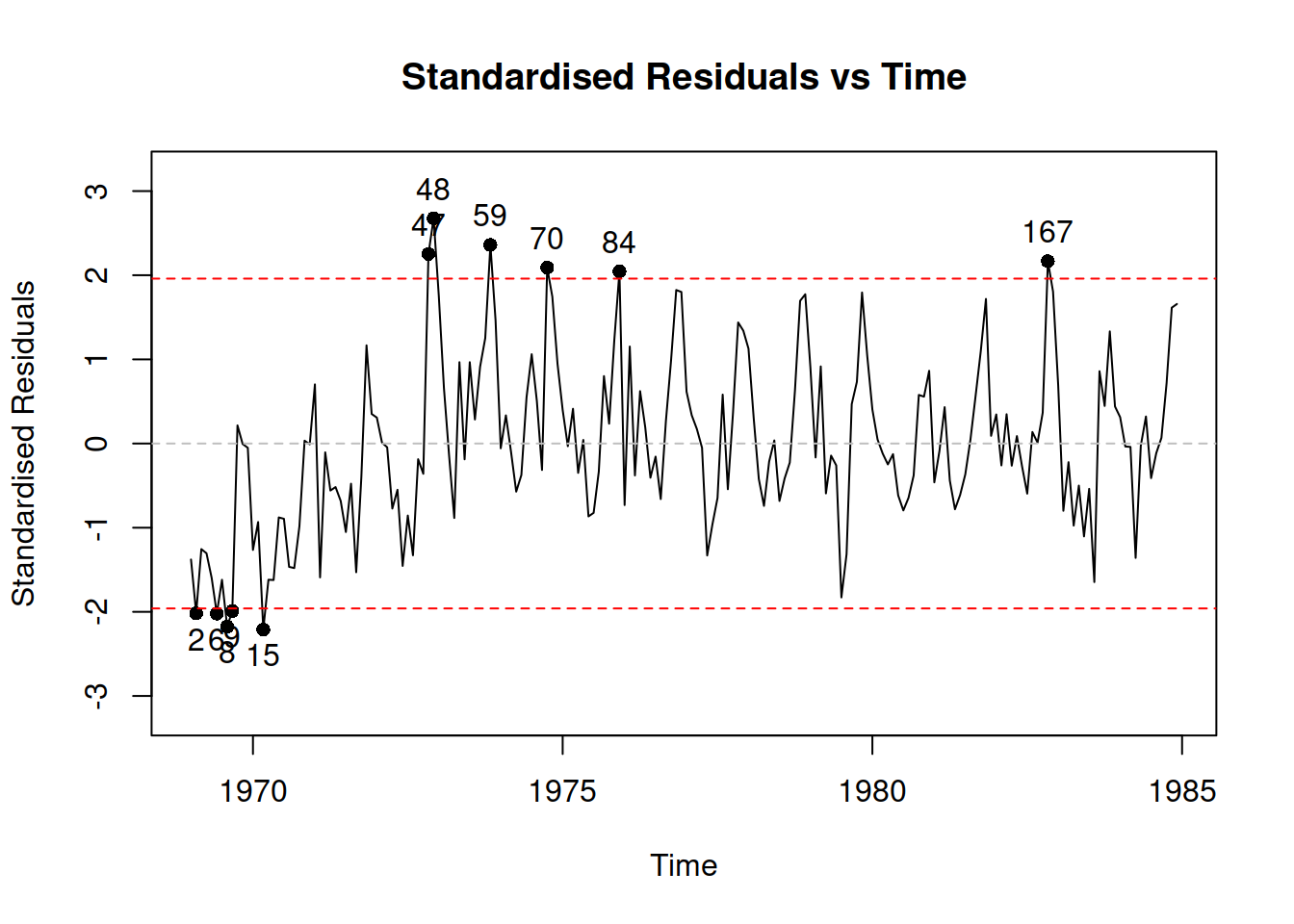Depending on the generated data, there might be issues in the ETS(M,Md,N) model for demand sizes, if the smoothing parameters are large. So, we can try out the ADAM logARIMA(1,1,2) to see how it compares with this model. Given that ARIMA is not yet implemented for the occurrence part of the model, we need to construct it separately and then use in adam():

oETSModel <- oes(y, "MMdN", h=10, holdout=TRUE,
occurrence=oETSModel,
orders=c(1,1,2),
distribution="dlnorm")
adamModeliARIMA
## Time elapsed: 0.1 seconds
## Model estimated using adam() function: iARIMA(1,1,2)
## Occurrence model type: Inverse odds ratio
## Distribution assumed in the model: Mixture of Bernoulli and Log Normal
## Loss function type: likelihood; Loss function value: 159.8986
## ARMA parameters of the model:
## AR:
## phi1
##  0.0955
## MA:
## theta1 theta2
##   -0.8503   -0.0573
##
## Sample size: 110
## Number of estimated parameters: 6
## Number of degrees of freedom: 104
## Number of provided parameters: 5
## Information criteria:
##      AIC     AICc      BIC     BICc
## 438.5901 439.4057 454.7930 456.7097
##
## Forecast errors:
## Asymmetry: 80.937%; sMSE: 157.584%; rRMSE: 0.594; sPIS: -4906.633%; sCE: 867.073%
plot(adamModeliARIMA,7)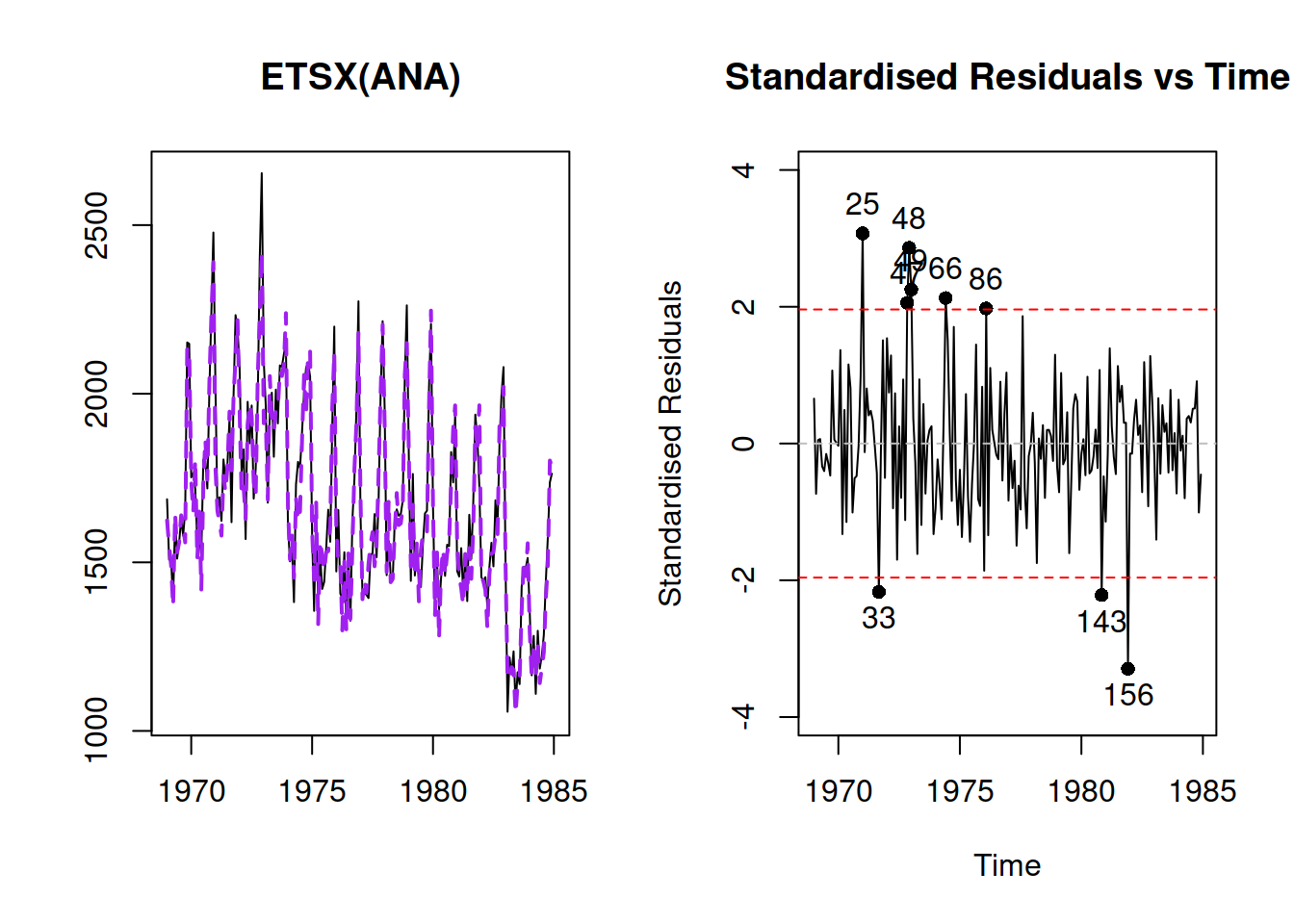Comparing the iARIMA model with the previous iETS based on AIC would not be fair, because as soon as the occurrence model is provided to adam(), he does not count the parameters estimated in that part towards the overal number of estimated parameters. In order to make the comparison fair, we need to estimate ADAM iETS in the following way:

adamModelsiETS[[i]] <- adam(y, "MMdN", h=10, holdout=TRUE,
occurrence=oETSModel)
adamModelsiETS[[i]]
## Time elapsed: 0.04 seconds
## Model estimated using adam() function: iETS(MMdN)
## Occurrence model type: Inverse odds ratio
## Distribution assumed in the model: Mixture of Bernoulli and Gamma
## Loss function type: likelihood; Loss function value: 151.26
## Persistence vector g:
## alpha  beta
##     0     0
## Damping parameter: 0.9902
## Sample size: 110
## Number of estimated parameters: 6
## Number of degrees of freedom: 104
## Number of provided parameters: 5
## Information criteria:
##      AIC     AICc      BIC     BICc
## 421.3130 422.1285 437.5159 439.4326
##
## Forecast errors:
## Asymmetry: 34.253%; sMSE: 89.82%; rRMSE: 0.448; sPIS: -2269.907%; sCE: 342.893%
plot(adamModelsiETS[[i]],7)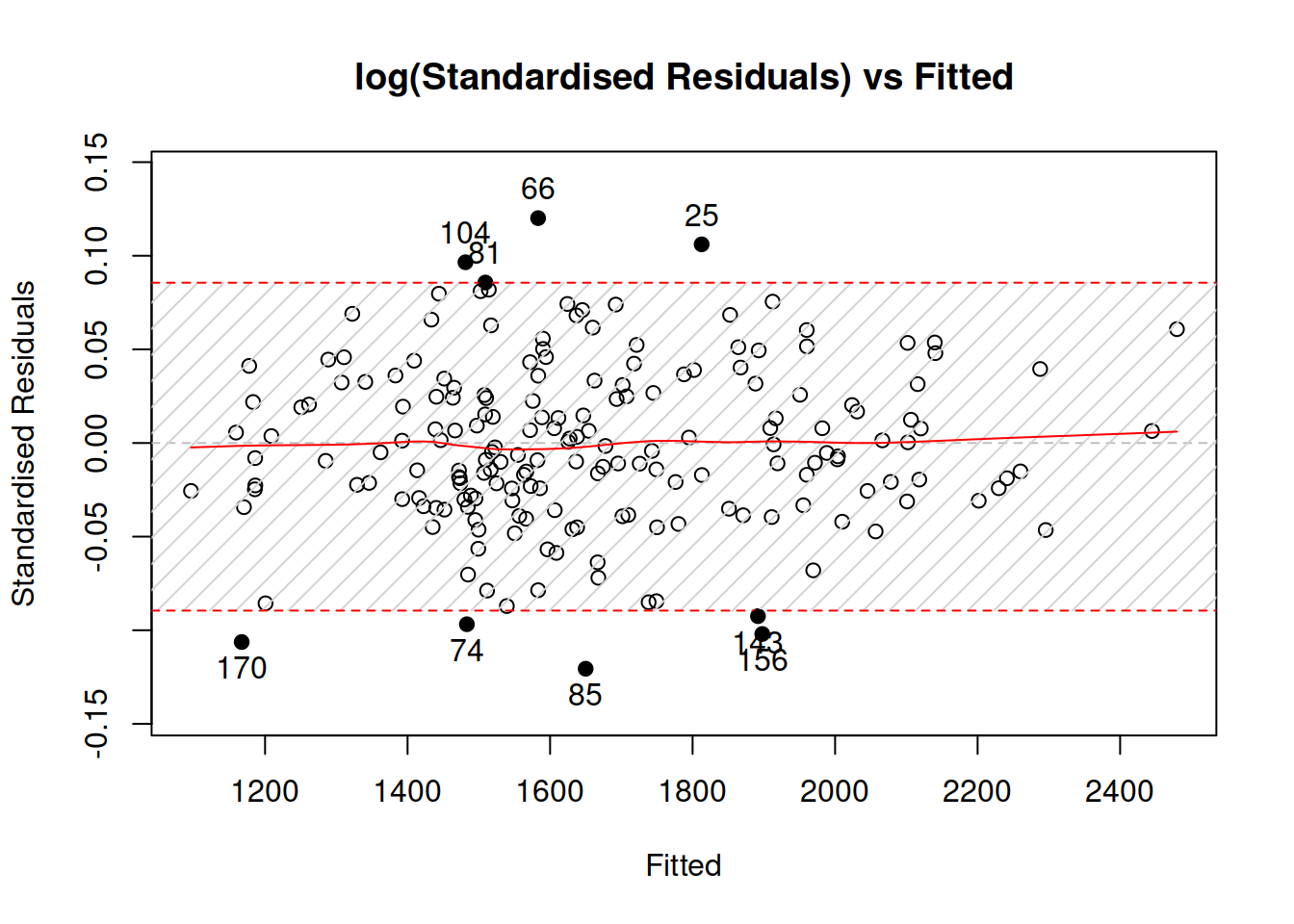Comparing information criteria, the iETS model is more appropriate for this data. But this might be due to a different distributional assumptions and difficulties estimating ARIMA model. If you want to experiment more with ADAM iARIMA, you might try fine tuning it for the data either by increasing the maxeval or changing the initialisation, for example:

adamModeliARIMA <- adam(y, "NNN", h=10, holdout=TRUE,
occurrence=oETSModel, orders=c(1,1,2),
distribution="dgamma", initial="back")
adamModeliARIMA
## Time elapsed: 0.09 seconds
## Model estimated using adam() function: iARIMA(1,1,2)
## Occurrence model type: Inverse odds ratio
## Distribution assumed in the model: Mixture of Bernoulli and Gamma
## Loss function type: likelihood; Loss function value: 175.7315
## ARMA parameters of the model:
## AR:
## phi1
## -0.2182
## MA:
## theta1 theta2
##   -0.4951   -0.2548
##
## Sample size: 110
## Number of estimated parameters: 4
## Number of degrees of freedom: 106
## Number of provided parameters: 5
## Information criteria:
##      AIC     AICc      BIC     BICc
## 466.2560 466.6369 477.0579 477.9532
##
## Forecast errors:
## Asymmetry: 57.886%; sMSE: 117.721%; rRMSE: 0.513; sPIS: -3639.472%; sCE: 623.486%
plot(adamModeliARIMA,7)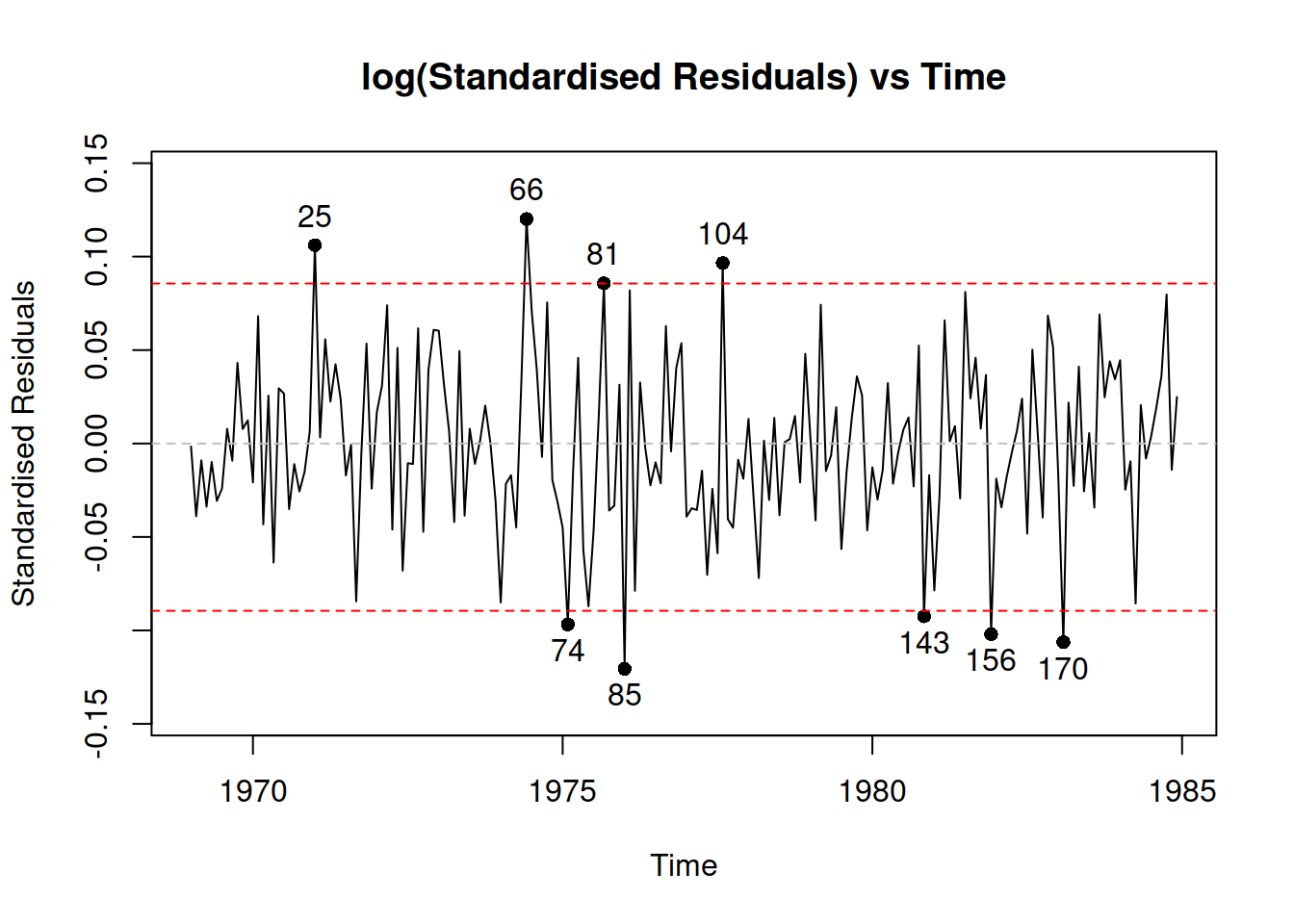Finally, we can produce point and interval forecasts from either of the model via the forecast() method. Here is an example:

adamiETSForecasts <- forecast(adamModelsiETS[[i]], h=10,
interval="prediction", nsim=10000)
plot(adamiETSForecasts)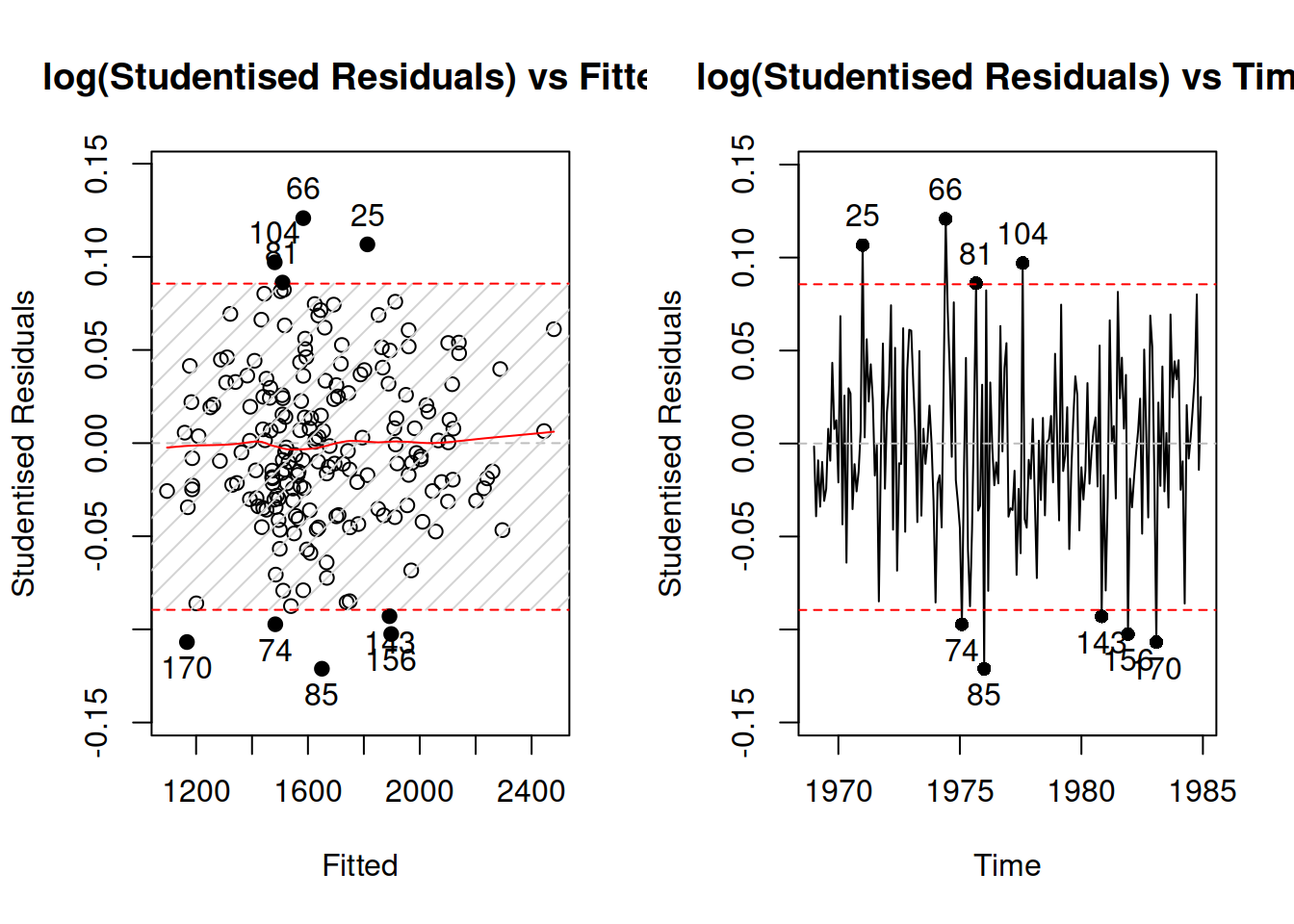The prediction intervals produced from multiplicative ETS models will typically be simulated, so in order to make them smoother you might need to increase nsim parameter, for example to nsim=100000.